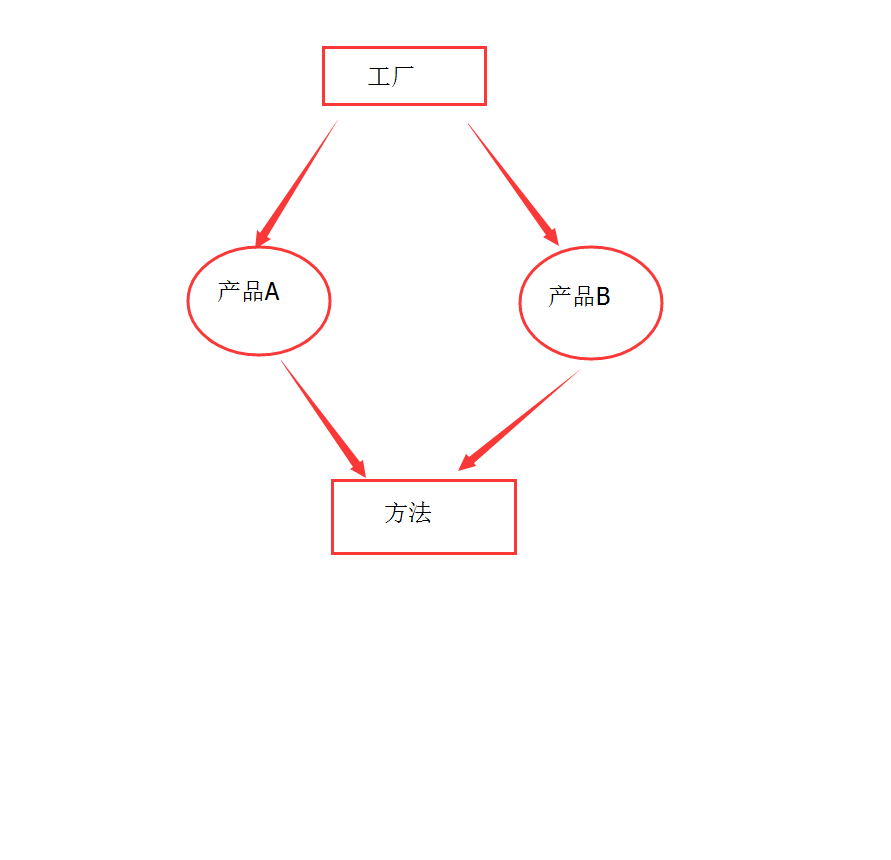# javascript设计模式之简单工厂模式

2017/04/17 21:28``````function productA(){
this.sum = 0
}
productA.prototype.create = function(sum){
this.sum +=sum
console.log("productA create: sum " + this.sum)
}
``````

``````function productB(name){
this.sum = 0
}
productB.prototype.create= function(sum){
this.sum +=sum
console.log("productB create: sum " + this.sum)
}``````

``````function factory(name){
switch (name){
case 'a':
return new productA()
break
case 'b':
return new productB()
break
}
}``````

``````var aFactory = factory("a")
var bFactory = factory("b")
aFactory.create(10) //打印 productA create: sum 10
bFactory.create(5) //打印 productB create: sum 5
aFactory.create(10) //打印 productA create: sum 20
bFactory.create(20) //打印 productB create: sum 25``````

0
0 收藏

### 作者的其它热门文章0 评论
0 收藏
0